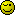# Fun mathematical puzzle

This puzzle makes me a headache…
If there is an array where is integer values in it (let’s say numbers: 1, 2, 3, 4, 5, 6, 7, 8, 9, 10, 11, 12, 13, 14, 15)

How you make a script what counts and shows all alternatives where four numbers sum(total) is example 40? One number can be use only one time.

Like example:
5 + 10 + 11+ 14 = 40
6 + 9 + 12 + 13 = 40
7 + 10 + 11 + 12 = 40
etc…

Quite puzzle, is it?Homework?

Tip: don’t add, subtract…Heh… no homeworkI can’t figure out how to loop it…

Give it your best shot, and post the code here. Then we can give you some clues on how to achieve this.

It is related to so called Knapsack problem.

I don’t have any idea how to do it…